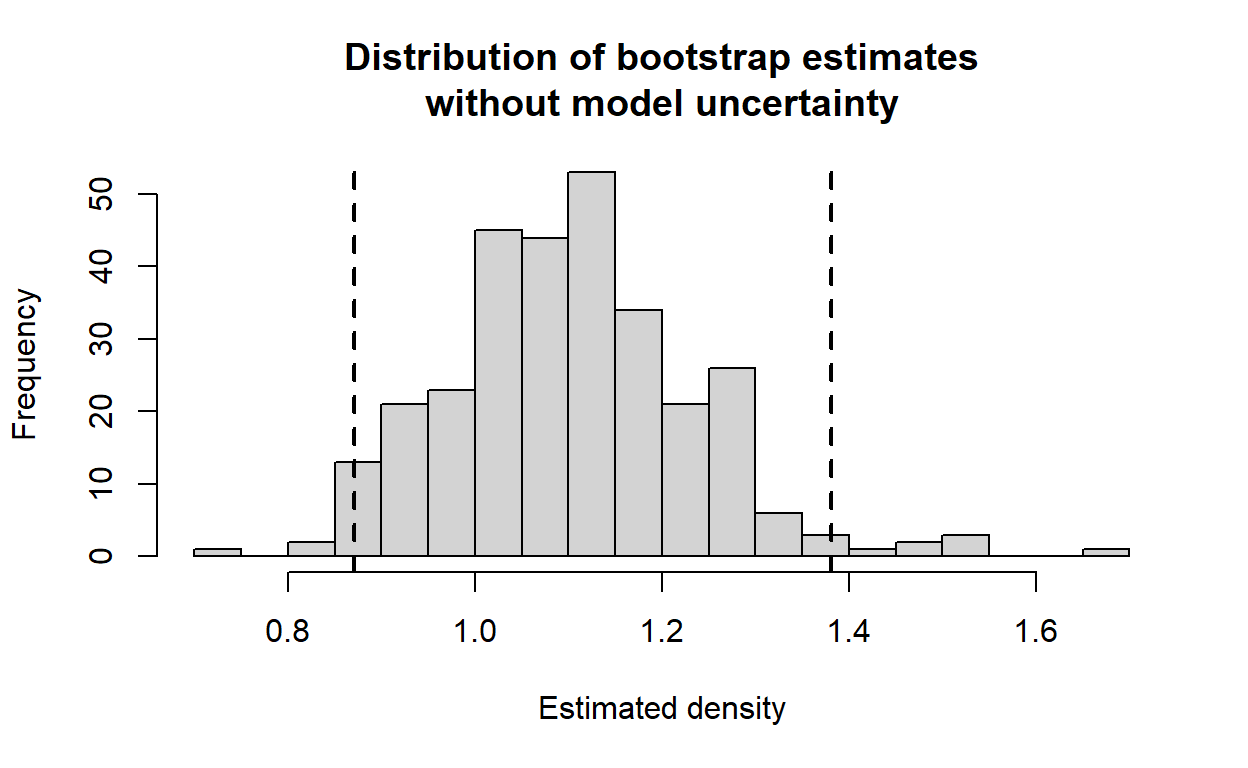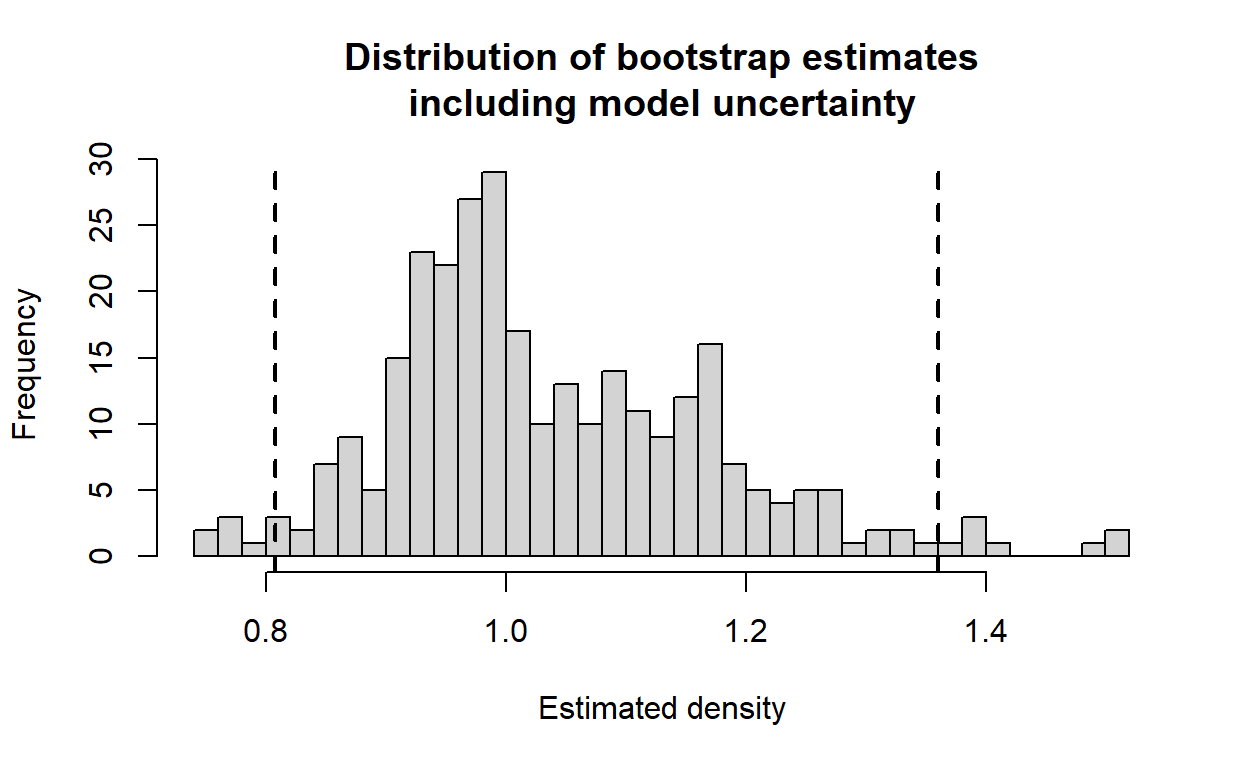# Variance estimation

Variance estimation using bootstrap resampling.

Eric Rexstad http://distancesampling.org (CREEM, Univ of St Andrews)https://creem.st-andrews.ac.uk
2022-02-22

Continuing with the Montrave winter wren line transect data from the line transect vignette, we focus upon producing robust estimates of precision in our point estimates of abundance and density. The analysis in R makes use of the Distance package .

# Objectives

• Estimate precision in the standard manner
• Use the bootstrap to estimate precision
• Incorporate model uncertainty in our estimates of precision

# Survey data

The R workspace wren_lt contains detections of winter wrens from the line transect surveys of S. T. Buckland (2006).

library(Distance)
data(wren_lt)


The function names() allows you to see the names of the columns of the data frame wren_lt. Definitions of those fields were provided in the line transect vignette.

The effort, or transect length has been adjusted to recognise each transect is walked twice.

conversion.factor <- convert_units("meter", "kilometer", "hectare")


# Fitting a suitable detection function

Rather than refitting models used in the line transect vignette, we move directly to the model selected by S. T. Buckland (2006).

wren.unif.cos <- ds(wren_lt, key="unif", adjustment="cos",
convert_units=conversion.factor)


Based upon experience in the field, the uniform cosine model was used for inference.

# Estimation of precision

Looking at the density estimates from the uniform cosine model

print(wren.unif.cos$dht$individuals$D)   Label Estimate se cv lcl ucl df 1 Total 1.067167 0.212552 0.1991741 0.7229762 1.575218 168.1373 The coefficient of variation (CV) is 0.199, and confidence interval bounds are (0.72 - 1.58) birds per hectare. The coefficient of variation is based upon a delta-method approximation of the uncertainty in both the parameters of the detection function and the variability in encounter rates between transects. $[CV(\hat{D})]^2 = [CV(\frac{n}{L})]^2 + [CV(P_a)]^2$ where • $$n$$ is number of detections • $$L$$ is total effort • $$P_a$$ is probability of detection given a bird is within the covered region. These confidence interval bounds assume the sampling distribution of $$\hat{D}$$ is log-normal . ## Bootstrap estimates of precision Rather than relying upon the delta-method approximation that assumes independence between uncertainty in the detection function and variability in encounter rate, a bootstrap procedure can be employed. Resampling with replacement of the transects produces replicate samples with which a sampling distribution of $$\hat{D}$$ is approximated. From that sampling distribution, the percentile method is used to produce confidence interval bounds respecting the shape of the sampling distribution . The function bootdht_Nhat_summarize is included in the Distance package. It is used to extract information from the object created by bootdht. I will modify it slightly so as to extract the density estimates rather than the abundance estimates. bootdht_Dhat_summarize <- function(ests, fit) { return(data.frame(D=ests$individuals$D$Estimate))
}


After the summary function is defined, the bootstrap procedure can be performed. Arguments here are the name of the fitted object, the object containing the data, conversion factor and number of bootstrap replicates. Here, I use the cores= argument to use multiple cores to process the bootstraps in parallel. If you do not have this many cores in your computer, you will need to reduce/remove the argument.

est.boot <- bootdht(model=wren.unif.cos, flatfile=wren_lt,
summary_fun=bootdht_Dhat_summarize,
convert_units=conversion.factor, nboot=300, cores=10)


The object est.boot contains a data frame with two columns consisting of $$\hat{D}$$ as specified in bootdht_Dhat_summarize. This data frame can be processed to produce a histogram representing the sampling distribution of the estimated parameters as well as the percentile confidence interval bounds.

alpha <- 0.05
(bootci <- quantile(est.boot$D, probs = c(alpha/2, 1-alpha/2), na.rm=TRUE))   2.5% 97.5% 0.8705481 1.3809942  hist(est.boot$D, nc=30,
main="Distribution of bootstrap estimates\nwithout model uncertainty",
xlab="Estimated density")
abline(v=bootci, lwd=2, lty=2)# Incorporating model uncertainty in precision estimates

The argument model in bootdht can be a single model as shown above, or it can consist of a list of models. In the later instance, all models in the list are fitted to each bootstrap replicate and model selection based on AIC is performed for each replicate. The consequence is that model uncertainty is incorporated into the resulting estimate of precision.

wren.hn <- ds(wren_lt, key="hn", adjustment=NULL,
convert_units=conversion.factor)
convert_units=conversion.factor)
est.boot.uncert <- bootdht(model=list(wren.hn, wren.hr.poly, wren.unif.cos),
flatfile=wren_lt,
summary_fun=bootdht_Dhat_summarize,
convert_units=conversion.factor, nboot=300, cores=10)

(modselci <- quantile(est.boot.uncert$D, probs = c(alpha/2, 1-alpha/2), na.rm=TRUE))   2.5% 97.5% 0.807331 1.360210  hist(est.boot.uncert$D, nc=30,
main="Distribution of bootstrap estimates\nincluding model uncertainty",
xlab="Estimated density")
abline(v=modselci, lwd=2, lty=2)